﻿ Right angle lever ( Trouton-Noble ) paradox

# Right-angle lever paradox shows special relativity is wrong.

## Relativistic momentum causes paradox.

(Fig.1) Four momentum.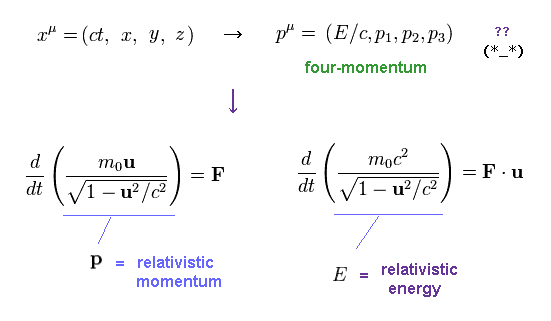Special relativity claims Four-momentum (= E/c, p ) transform like spacetime ( ct, x, y, z ) under Lorentz transformation.
"E" and "p" are relativistic momentum and energy, respectively.

Newtonian force F is defined as the rate of change of momentum (= p ) with respect to time.
And the time derivative of energy (= E ) is equal to force F × velocity (= u ).

## Force Fy is reduced by observer's movement.

(Fig.2) Newtonian force Fy is reduced when it is moving.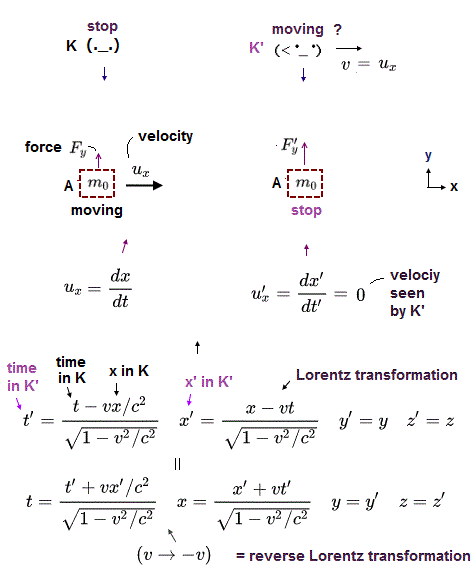For relativistic energy and momentum to satisfy the equations of Fig.1, force Fy must decrease when it is moving.
See various sites ( this (v=0), this (p.3), this (p.4,5), this (p.15) ).

In Fig.2 left, an observer (= K ) and an object (= M ) are at rest, and force Fy is applied to this object in y direction.

When the observer starts to move in x direction at velocity "v", the object is moving in the opposite direction from his viewpoint (= K', Fig.2 right ).
Force in y direction in K' frame is reduced according to special relativity.

## Two equal forces Fy - one is at rest, another is moving.

(Fig.3) Two equal forces Fy are in equilibrium.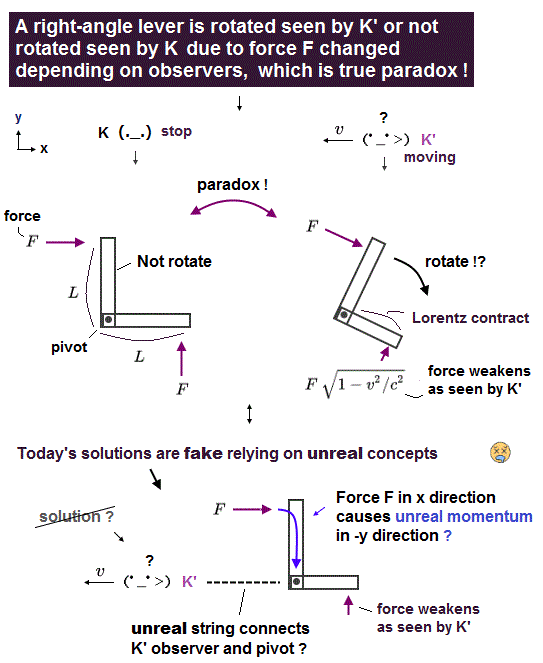In Fig.3, two equal forces Fy are applied to the stationary lever in K frame (= observer at rest ).
This lever has two equal arms on both sides of a fulcrum, so it is in balance, NOT rotate in K frame.

One of these forces (= Fig.3 left ) is at rest, and another force ( source ) is moving at velocity "v" in x direction.

## Lever rotates Only in K' frame !

(Fig.4) Lever rotates from the viewpoint of moving observer (= K' ) ?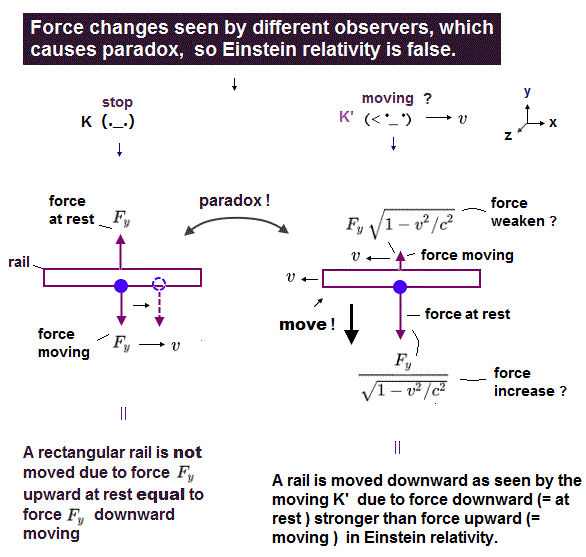When the observer is moving at "v" in x direction (= K' ), this lever and left force start to move in the opposite direction.
As a result, this force (= Fig.4 left ) is reduced, as shown in Fig.2.

On the other hand, right force looks stationary in K' frame, it is increased by observer's movement.
As a result, this lever rotates clockwise only in K' frame in special relativity !

This unreasonable transformation of force Fy is one of causes of right angle lever paradox below.
Of course, this paradox has NO solutions, which means relativistic momentum and energy are wrong.

## Observer can rotate lever without touching it !

(Fig.5) Special relativity gives observer "supernatural power" ?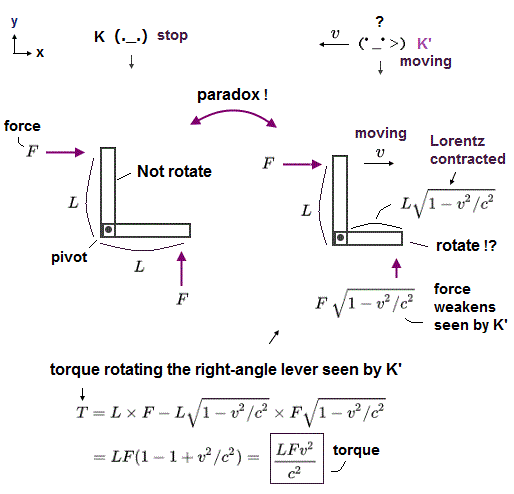Here is a right-angle lever with two arms which can rotate around a stationary point.
In Fig.5 left, two arms of this lever are equal in the length.

And the forces F acting on them are completely the same, which directions are perpendicular to each other.
So in Fig.5 left, this lever is at rest ( not rotating ) due to equilibrium of forces. ( torque: F×L = F×L. )

But when the observer starts to move, this lever starts to rotate, though he doesn't touch it ! (= Fig.5 right. )
This is clearly a fatal paradox, and shows special relativity is wrong.

## Why "balanced" lever becomes "unbalanced" by observer's movement ?

(Fig.6) Why the lever "rotates" just by observer's movement ?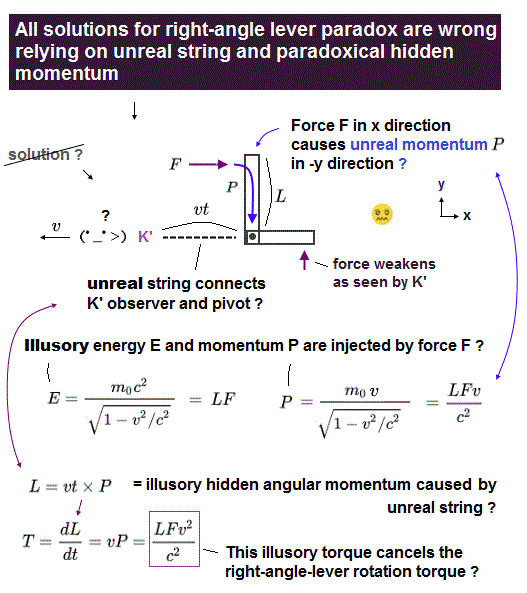Here we explain why this right-angle lever rotates just by simple observer's movement.
One arm (A-2) is Lorentz-contracted in the moving direction. ( = x direction )

And furthermore, the force F, which is perpendicular to the moving direction, becomes weaker than the orginal F according to the special relativity, by a factor of (1-β2)1/2.

As a result, torque of the lever is NOT zero only from the viewpoint of moving observer.
From the stationary observer, the lever torque is zero. This is paradox.

## Lorentz transformation of Newtonian forces in x, y directions.

(Fig.7) Trasformation of Newtonian forces in x, y directions.The force in x direction remains the same ( F'x = Fx ), and the force in y direction is reduced ( F'y < Fy ) under Lorentz transformation, as shown in various sites ( this (v=0), this (p.3), this (p.4,5), this (p.15) ).

In Fig.7, Lorentz transformation is in x direction.
And "rest" means the force applied to a object at rest.

## Newtonian force Fx doesn't change under Lorentz transformation.

(Fig.8) Force parallel to observer's movement is invariant   ( Fx = F'x )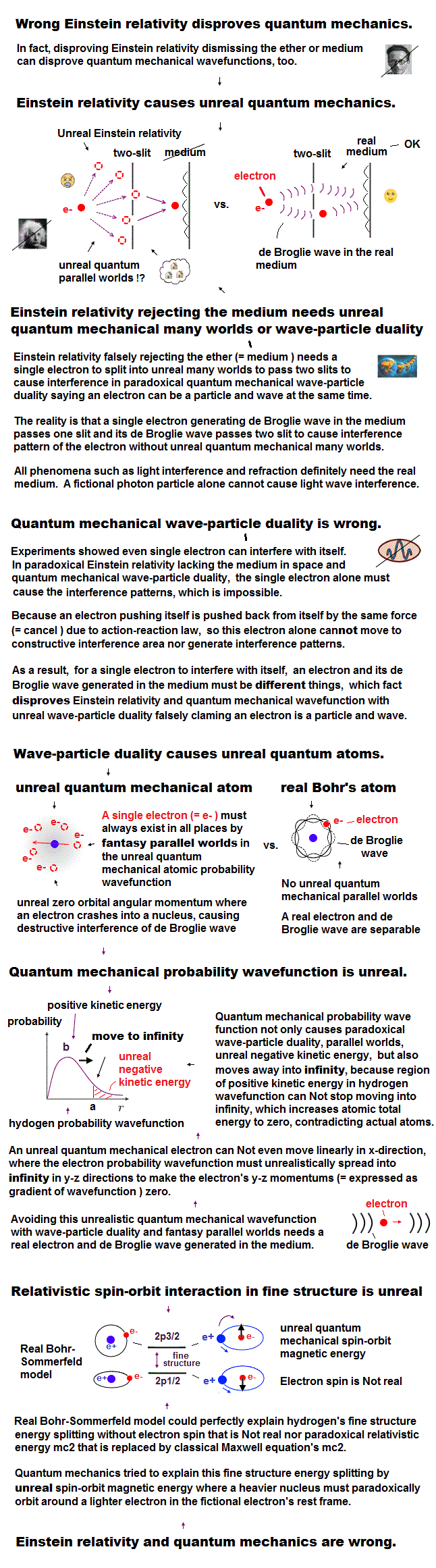In Fig.8, an object (= M ) is moving at velocity u = "v" in x direction relative to the stationary observer in K frame.
Force Fx (= ordinary force, NOT four-force ) is applied to this object in K.

As seen by the observer moving at velocity "v" in x direction (= K' ), this object looks stationary.
So, K' frame is the rest frame of the object.

The force Fx transforms to F'x in K' frame.
According to special relativity, these forces are equal ( Fx = F'x ) in any frames.

## Newtonian force Fy is reduced under Lorentz transformation.

(Fig.9) Force perpendicular to observer's movement decreases   ( Fy < F'y )On the other hand, force (= Fy ) perpendicular to the observer's movement is reduced in K frame than the force (= F'y ) in the rest frame (= K' ) of the object.

As seen by the moving observer in K' frame, the object is at rest, and force F'y is applied (= Fig.9 right ).
From the perspective of stationary observer in K frame, the object is moving, and its force decreases ( Fy < F'y ).

## Lorentz transformation.

(Eq.1) Lorentz transformation of space-time coordinate.According to special relativity, the space-time coodinate (= t, x, y, z ) transform, obeying Lorentz transformation.

## Four-momentum.

(Eq.2)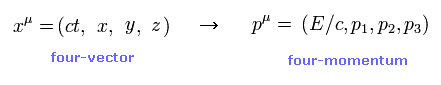Special relativity claims Four-momentum transform like spacetime ( ct, x, y, z ) under Lorentz transformation. See this (p.15) and this (p.12)

This transformation of relativistic energy (= E ) and momentum (= p ) is used in Dirac equation, as shown on this page.
Above transformation of Newtonian force is indispensable for this four-momentum relation.

## Lorentz transformation of four-momentum.

(Eq.3)So, the momentum (= p'x ) in K' frame is given by Eq.3.
Relativistic momentum (= px ) in x direction and energy (= E ) in K are mixed.

(Eq.4) From the viewpoint of "stationary" observer (= K ).From the viewpoint of stationary observer (= K ), an obejct is moving at velocity u (= v ) to the right.
Force (= F ) is equal to the rate of change in momentum (= p ).

The rate of change in energy (= E ) is equal to the force × velocity "u".
Because the force × moving distance is energy E.

(Eq.5) From the viewpoint of moving observer (= K' )In K' frame, the observer is moving at velocity "v" to the right.
The object is moving in the same direction, so it looks stationary as seen by the observer in K'.

So the velocity of the object in K' is u' = 0.
The momentum (= p' ) and energy (= E' ) are given by the relation of Eq.3.

About the calculation of Lorentz transformation of forces in each direction, see this section

## Calculating torque in each frame.

(Eq.6) Lever is at rest in K' frame, but rotates only in K frame.A right angle lever is moving at velocity u = v in x direction.
The observer in K' frame is also moving at the same velocity v in x direction.

(Eq.7) Lever is at rest in K' frame (= moving observer ).So this lever is stationary in K' frame (= rest frame of the lever ).
We suppose the lengths of the lever arms and the applied forces are equal in K' frame.

As a result, the lever is in equilibrium, and does NOT ratate (= torque is zero ) in K' frame.

(Eq.8) Lever rotates ONLY in K frame.   Torque in K frame is NOT zero.From the perspective of stationary observer in K frame, this lever is moving to the right.
The lever arm parallel to the moving direction is Lorentz contracted.

And the Newtonian force Fy is reduced, compared to F'y at rest frame.
As a result, torque τ is NOT zero, as shown in Eq.8.

## Solution to this paradox depends on "fake" center.

(Fig.10) Strange energy flows inside lever ?   Virtual center of rotation !?To solve this right-angle lever paradox, they introduced unrealistic concepts such as "virtual" center of rotation, and energy flow, as shown on this site and this site.

"p" is also "virtual" momentum flowing through the lever.
They suppose virtual center of rotation exists at the point of observer.

(Fig.11) "Virtual" torque is NOT real.Torque is given by the force times radius.
But they changed this definition, as shown in Fig.11 lower and this page.

They introduced virtual center of rotation at oberver.
So this ( virtual ) radius "r" inreases as r = vt ( "v" is velocity ).
Torque can be expressed by derivative of this radius "r" × fake momentum p.

In Fig.10, the lever is moving at speed "v" with respect to K frame in the x direction.
So they supposed the external force Fx do work "Fv" per second on the point "1" of the lever.

(Eq.9)   ↓ Virtual energy flow.And they supposed this strange energy flows to the point "A" at the speed of "U" inside the lever.
In Eq.9, T is the time needed for this energy to reach the point "A".
And E is the accumulated energy during this time T inside the whole arm of "1-A" .

(Eq.10)   ↓ "Virtual" accumulated energy inside arm.Using the relation between relativistic energy "E" and momentum "p", Eq.10 is the momentum accumulated in the arm "1-A".
( Relativistic momentum p = energy E × U/c2.   U is velocity of virtual energy. )

And they artificially supposed this energy flows outside from the pivot "A" due to the law of action and reaction.

## "Fake" torque based on virtual center.

(Eq.11) ↓ "Fake" torque.And again they used "ad-hoc" idea that the observer of K frame is the center of rotation, though this observer is NOT connected to this lever arm by any string or forces.

They utilize the fact that torque is given by the time derivative of the angular momentum.
But they artificially replaced the meaning of the torque by other unreal concepts, as shown in Fig.11.

## Accidental coincidence in torque ?

(Fig.12) Torque based on "virtual" center coincides with "real" torque !?The distance between the lever and observer is "vt", so the new angular monentum is L = "vtp".
So the time derivative of this L becomes "vp", they insist.

As a result, the torque τ = "vp" (= Eq.11 ) becomes just equal to Eq.8, and they insisted the lever torque is used in the strange energy flow and no rotation happens.

## Virtual energy flow inside arm is illusion.

(Fig.13) Force F does NOT do work on point 1.But this method depends on some unreasonable assumptions.
First, force Fx does NOT do work on the point "1", when the lever torque is zero ( or weakened ).
( This force Fx is cancelled out by the force Fy because of rigid lever. )

For example, we suppose the lever at rest is in equilibrium due to equal forces (= F ) applied to two arms.
( In this case, force F does NOT work on this lever, because the lever is neither moving nor rotating. )

But, when the observer starts to move to the left, this lever starts to move to the right from the observer's perspective.
Based on the above "ad-hoc" assumption, this force F does work just by observer's movement !
Of course, it is impossible.

## Right angle lever paradox has NOT been solved.

(Fig.14) Lever rotates "and" does NOT rotate at the same time ?   ← Paradox !And as you notice, this energy flow inside the lever and the strange center of rotation are NOT true.
In conclusion, right-angle lever paradox has NOT been solved and shows the relativistic theories including QED, and standard moded are all wrong.

## "Acceleration" cannot solve this paradox.

(Eq.12) Relativistic momentum p.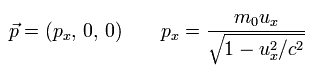In this paper, they tried to solve this right angle lever paradox using different relativistic mass in x, y directions.

Suppose a object with rest mass (= m0 ) is moving at velocity ux in x direction.
So the relativistic momentum is given by Eq.12.

(Eq.13) Relativistic mass in x directionUsing the momentum of Eq.12, we obtain the relativistic mass in x direction, as shown in Eq.13.
Here we use
(Eq.14)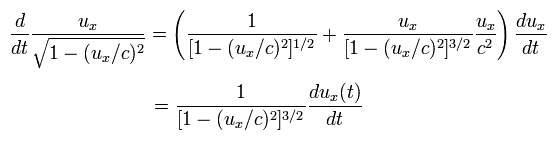In the same say, the relativistic mass in y direction becomes

(Eq.15) Relativistic mass in y direction.From Eq.13 and Eq.15, you find the relativistic mass in x direction is heavier than that in y direction.

(Eq.16) Mass in x direction is heavier than y direction.From Eq.13, the acceleration (= ax ) in the x direction is
(Eq.17)From Eq.15, the acceleration (= ay ) in the y direction is
(Eq.18)So the acceleration is bigger in y direction than x direction due to the difference of relativistic masses between x and y directions.

## Torque becomes zero ? ← But this solution is wrong.

(Eq.19) Torque = acceleration ( instead of force ) × length ?They use acceleration instead of force, when calculating torque.
From Eq.17, Eq.18, Eq.19, the torque in K frame (= lever moving frame ) becomes
(Eq.20)As a result, also in K frame, the torque is zero. So there is no right-angle lever paradox, they insist.
But is it really so ?
Eq.17 and Eq.18 use the difference between the relativistic masses in the x and y direction.

The relativistic mass in the x direction is heavier than that in the y direction, so the acceleration is bigger in the y direction.
This cancels out the torque ?
In fact, the relativistic masses and accelerations are the same in both cases, because of "rigid" body.

## "Total" relativistic mass of lever is the same in both directions !

(Fig.15) Relativistic masses are " equal " in both cases.In Fig.15 left, the force Fy is trying to rotate the whole lever clockwise.
In this case, the 1-A arm is accelerated in the x direction, and the A-2 arm is accelerated in the y direction.
So this rotation contains different relativistic masses.

In Fig.9 right, the force Fx is trying to rotate the whole lever counterclockwise.
Also in this case, the 1-A arm is accelerated in the x direction, and the A-2 arm is accelerated in the y direction.

## Right- angle lever paradox has NOT been solved.

As a result, both these two rotations ( due to Fx and Fy ) contain same relativistic mass.
So the torque = "force × length" is equal to "acceleration × length", which situation does NOT change.

This means idea of acceleration cannot stop rotation of this lever.
This right-angle lever remains a fatal paradox of special relativity.

## Transformation of forces.

(Ap.1)   K' and object are moving at v (= u ) in x direction.K' frame and the object are moving at velocity u = v in x direction, relative to K frame.
Momentum and energy of the object as seen by observers in K and K' frames are ( p, E ) and ( p', E' ), respectively

Defferentiating p'x (= momentum in K' ) with respect to the time t (= K ), we get Ap.1.
Here we use transformation of Ap.2 and the definition of Ap.3.

(Ap.2) Lorentz transformation of four-momentum.(Ap.3) From the viewpoint of different observers ( K and K' ).Using Lorentz transformation of the time, and differentiating t' with respect to t, we obtain
(Ap.4)"ux" is the object's velocity in x direction from the viewpoint of K frame.
Using Ap.4 and comparing the result with Ap.1, we get
(Ap.5)Here, the object is moving in x direction at u = v in K frame, so we get the relation of

(Ap.6)   Force in x direction does NOT change. ( Fx = F'x )F'x is the force in x direction acting on the object in K' frame (= rest frame of the object ).
According to the special relativity, force in x direction is invariant in any frames. ( F'x = Fx )

## Force in y direction decreases ( F'y > Fy )

(Ap.7)   Transformation of force in y direction.Using Ap.2, Ap.3, Ap.4, we obtain Ap.7 in force in y direction.

(Ap.8)   Force in y direction is reduced. ( F'y > Fy )As shown in Ap.8, the force (= Fy ) in y direction is reduced compared to force (= F'y ) of rest frame.
This is one of reasons why right angle lever paradox happens.

## Four-force, four-velocity.

(Ap.9)   Ordinary velocity (= u ) from stationary observer in K frame.In this section, we explain transformation of forces with respect to relativistic four-forces.
In Ap.9, an object is moving at velocity "u" relative to the stationary observer (= K frame ).

## Proper time τ is NOT real.

(Ap.10)   Lorentz invariant proper time (= τ )In relativistic kinematics, we often use the convenient proper time, which is Lorentz invariant.
So, this proper time is NOT real "time" in each frame.

They utilize this proper time ONLY for making equation of motion Lorentz-covariant.
That's all.

(Ap.11)From Ap.11, we can prove this proper time τ has a Lorentz-invariant form.

From Ap.11, we have
(Ap.12)In relativistic world, the ordinary velocity (= u ) transforms very complexly.
Because, both the time (= dt ) and space (= dx ) coordinates change.

So they tried to use convenient four-velocity (= ω ), as shown on this (p.111) and this site.

(Ap.13)   Ordinary velocity (= u ) and four-velocity (= ω )The denominator of this four-velocity ω is Lorentz-invariant proper time.
So, only its numerator (= dx, dy, dz, cdt ) transforms under Lorentz transformation.

(Ap.14)   0-component of this four-velocity0-component of four vector is "ct".
So subsituting "ct" and Ap.11 into Ap.14, we can get the zeroth-component of this four-velocity.

(Ap.15)   Relativistic equation of motion.They define relativistic equation of motion like Ap.15.
The left side is derivative of four velocity with respect to proper time.

So again, this transforms like four-vector. See this (p.7) and this site.
The right side (= f ) of Ap.15 is called "four-force", very artificial concept.

(Ap.16)   Four-force f,   ordinary force F.On this page, "F" denotes ordinary ( Newtonian ) force, and "f" denotes four (vector) force.
This four-force transforms like four-vector, which is NOT a real force.

Using the proper time of Ap.10, we have
(Ap.17)Substituting Ap.13, Ap.16 and Ap.17 into the relativistic equation of motion (= Ap.15 ), we get the relation of Ap.18.

(Ap.18)If we define relativistic momentum p as
(Ap.19)Ap.18 becomes
(Ap.20)Ap.20 is ordinary equation of motion.
Only "p" must be relativistic momentum like Ap.19.

(Ap.21)For these four-forces to transform as four-vector, 0-component of four-vector must have the form of Ap.21.
Substituting Ap.14 and Ap.21 into Ap.15, we have

(Ap.22)Here we define relativistic energy of
(Ap.23)## From four-force to Newtonian force.

An object is moving at velocity u = v in x direction in K frame, so

(Ap.24)Substituting Ap.24 into Ap.16 and Ap.21, we have
(Ap.25)Four-force transform like four-vector ( ct, x, y, z ) under Lorentz transformation.
Using 0 and x components of Ap.25, we obtain

(Ap.26)In K' frame, the object is at rest, so the velocity (= u' ) in K' is zero.
So, four-force in K' frame is equal to the ordinary Newtonian force.

(Ap.27)From Ap.26 and Ap.27, we find Newtonian force in x direction is invariant under Lorentz transformation.
On the other hand, four-vector force fy doesn't change under Lorentz transformation, so

(Ap.28)We can get the same result of Ap.8.2014/6/1 updated. Feel free to link to this site.